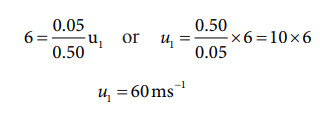Home | | Physics 11th std | Perfect inelastic collision

# Perfect inelastic collision

Let the two bodies with masses m1 and m2 move with initial velocities u1 and u2 respectively before collision.

Perfect inelastic collision

In a perfectly inelastic or completely inelastic collision, the objects stick together permanently aft er collision such that they move with common velocity. Let the two bodies with masses m1 and m2  move with initial velocities u1 and u2 respectively before  collision. Aft er perfect inelastic collision both the objects move together with a common velocity v as shown in Figure (4.17).

Since, the linear momentum is conserved during collisions,

m1u1 + m2u2 = (m1 + m2)vThe common velocity can be computed by## Solved Example Problems for Perfect inelastic collision

### Example 4.21

A bullet of mass 50 g is fired from below into a suspended object of mass 450 g. The object rises through a height of 1.8 m with bullet remaining inside the object. Find the speed of the bullet. Take g = 10 ms-2.

### Solution

m1 = 50 g = 0.05 kg; m2 = 450 g = 0.45kgThe speed of the bullet is u1. The second body is at rest u2 = 0 . Let the common velocity of the bullet and the object after the bullet is embedded into the object is v.The combined velocity is the initial velocity for the vertical upward motion of the combined bullet and the object. From second equation of motion,Substituting this in the above equation, the value of u1 isStudy Material, Lecturing Notes, Assignment, Reference, Wiki description explanation, brief detail
11th Physics : UNIT 4 : Work, Energy and Power : Perfect inelastic collision |